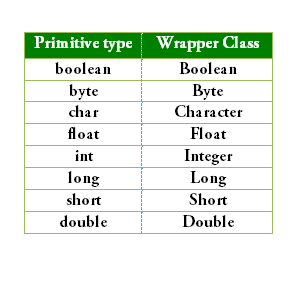Open In App
Related Articles
• Write an Interview Experience
• Java Tutorial
• Java Collection Tutorial

# Need of Wrapper Classes in Java

Firstly the question that hits the programmers is when we have primitive data types then why does there arise a need for the concept of wrapper classes in java. It is because of the additional features being there in the Wrapper class over the primitive data types when it comes to usage. These methods include primarily methods like valueOf(), parseInt(), toString(), and many more.

A wrapper class wraps (encloses) around a data type and gives it an object appearance. Wrapper classes are final and immutable. Two concepts are there in the wrapper classes namely autoboxing and unboxing.

Autoboxing is a procedure of converting a primitive value into an object of the corresponding wrapper class. For example, converting int to Integer class. The Java compiler applies autoboxing when a primitive value is:

• Passed as a parameter to a method that expects an object of the corresponding wrapper class.
• Assigned to a variable of the corresponding wrapper class.

Unboxing is a procedure of converting an object of a wrapper type to its corresponding primitive value. For example conversion of Integer to int. The Java compiler applies to unbox when an object of a wrapper class is:

• Passed as a parameter to a method that expects a value of the corresponding primitive type.
• Assigned to a variable of the corresponding primitive type.

Autoboxing and unboxing are pictorially depicted below:Now let us land on discussing the useful features of wrapper classes, they are listed as follows:

1. They convert primitive data types into objects. Objects are needed if we wish to modify the arguments passed into a method (because primitive types are passed by value).
2. The classes in java.util package handles only objects and hence wrapper classes help in this case also.
3. Data structures in the Collection framework, such as ArrayList and Vector, store only objects (reference types) and not primitive types.
4. An object is needed to support synchronization in multithreading.

One of the major important features provided by wrapper classes is a lot of utility methods. Say when we have a float value, and we want to find the integer value of that float, then we have a specific method for that which is depicted from the illustration given below.

Illustration:

If we want to create an integer value from a string or a boolean value from a string. We can do it with the help of wrapper classes.

Syntax: Creation from other data types

```Integer hundred = Integer.valueOf("100");
Boolean value = Boolean.valueOf("True");```

Simple Autoboxing of wrapper class example

## Java

 `import` `java .util.*;``class` `gfg {` `    ``public` `static` `void` `main(String args[]) {``        ``int` `x = ``5``;``        ``float` `y = ``3``.14f;``        ``long` `z=``6000``;` `        ``// autoboxing``        ``Integer intobj = x;``        ``Float floatobj=y;``        ``Long longobj=z;``        ``// print``        ``System.out.println(intobj);``        ``System.out.println(floatobj);``        ``System.out.println(longobj);``    ``}``}`

Output

```5
3.14
6000```

Example:

## Java

 `// Java Program to Show Wrapper class concept` `// Importing input output classes``import` `java.io.*;` `// Main Class``class` `GFG {` `    ``// Main driver method``    ``public` `static` `void` `main(String[] args)``    ``{` `        ``// In java, in case of floating values``        ``// they are stored as x = (y)f` `        ``// Conversion of float value to int``        ``Float floatWrap = Float.valueOf(``45``.158f);` `        ``// Invoking the intValue() method over the stored``        ``// float value to store``        ``int` `floatToInt = floatWrap.intValue();` `        ``// Print the non-primitive(Integer) value``        ``System.out.println(floatToInt);` `        ``// Now for another number N``        ``// Say N = 5` `        ``// Convert the binary number to the integer value``        ``Integer five = Integer.valueOf(``"101"``, ``2``);` `        ``// Print the number``        ``System.out.println(five);``    ``}``}`

Output

```45
5```